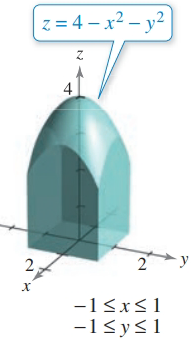Chapter 7.9, Problem 19E### Calculus: An Applied Approach (Min...

10th Edition
Ron Larson
ISBN: 9781305860919

#### Solutions

Chapter
Section### Calculus: An Applied Approach (Min...

10th Edition
Ron Larson
ISBN: 9781305860919
Textbook Problem
1 views

# Finding the Volume of a Solid Region In Exercises 13-20, use a double integral to find the volume of the solid region.To determine

To calculate: The volume of solid region that shown below,Explanation

Given information:

The provided solid region is

Formula used:

The procedure to calculate volume of surface z=f(x,y),

Step-1: Write the equation of surface in the form z=f(x,y)

Step-2: Sketch the projected region R in the x-y-plane.

Step-3: Determine the order of limits of integration.

Step-4: Evaluate the volume of solid region,

Volume=mnabf(x,y)dxdy

Here, the projected region is R:

myn and axb

Integration property aaf(x)dx=20af(x)dx for every even function f(x).

Calculation:

Consider graph of solid region,

The equation of upper surface written as follow.

z=4x2y2

The projected region R: 1x1,1y1 in the xy-plane written as follow,

The bounds for x are 1x1 and bounds for y are 1y1.

The volume for the solid region is,

Volume=1111(4x2y2)dxdy

Now apply, integration property aaf(x)dx=20af(x)dx for every even function f(x)

### Still sussing out bartleby?

Check out a sample textbook solution.

See a sample solution

#### The Solution to Your Study Problems

Bartleby provides explanations to thousands of textbook problems written by our experts, many with advanced degrees!

Get Started

#### Find more solutions based on key concepts